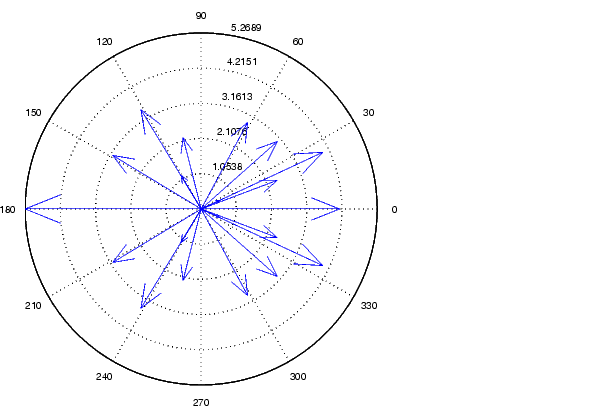MATLAB Function Referencecompass

Plot arrows emanating from the origin

Syntax

• ```compass(U,V)
compass(Z)
compass(...,`LineSpec`)
compass(axes_handle,...)
h = compass(...)
```

Description

A compass graph displays the vectors with components `(U,V)` as arrows emanating from the origin. `U`, `V`, and `Z` are in Cartesian coordinates and plotted on a circular grid.

```compass(U,V) ``` displays a compass graph having n arrows, where n is the number of elements in `U` or `V`. The location of the base of each arrow is the origin. The location of the tip of each arrow is a point relative to the base and determined by `[U(i)`,`V(i)]`.

```compass(Z) ``` displays a compass graph having n arrows, where n is the number of elements in `Z`. The location of the base of each arrow is the origin. The location of the tip of each arrow is relative to the base as determined by the real and imaginary components of `Z`. This syntax is equivalent to `compass(real(Z),imag(Z))`.

```compass(...,LineSpec) ``` draws a compass graph using the line type, marker symbol, and color specified by `LineSpec`.

```compass(axes_handle,...) ``` plots into the axes with handle `axes_handle` instead of the current axes (`gca`).

```h = compass(...) ``` returns handles to line objects.

Examples

Draw a compass graph of the eigenvalues of a matrix.

• ```Z = eig(randn(20`,`20));
compass(Z)```

See Also

`feather`, `LineSpec`, `quiver`, `rose`

Direction and Velocity Plots for related functions

Compass Plots for another example

© 1994-2005 The MathWorks, Inc.